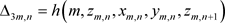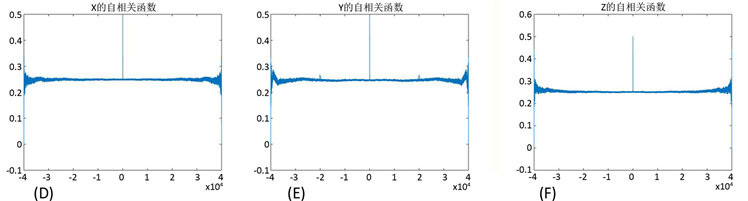﻿ 基于拉丁方与时变符号混沌系统的流密码算法设计

# 基于拉丁方与时变符号混沌系统的流密码算法设计Design of a Stream Cipher Algorithm Based on Latin Square and Time-Varying Symbolic Chaotic System

Abstract: This paper studies chaos of a class of three-dimensional time-varying generalized symbolic systems, and analyzes the pseudo-randomicity of its solutions. Then, combined with the basic cryptosystem designed by Latin square, a stream cipher algorithm was designed. Finally, the encryption effect of the algorithm on digital image is simulated and compared with the simulation results of the modulo 2 addition stream cipher system. Simulation shows that this algorithm has good encryption effects.

1. 引言

$\left\{\begin{array}{l}{x}_{m+1,n}=f\left(m,{x}_{m,n},{y}_{m,n},{z}_{m,n},{x}_{m,n+1}\right)\\ {y}_{m+1,n}=g\left(m,{y}_{m,n},{x}_{m,n},{z}_{m,n},{y}_{m,n+1}\right)\\ {z}_{m+1,n}=h\left(m,{z}_{m,n},{x}_{m,n},{y}_{m,n},{z}_{m,n+1}\right)\end{array}$ (1)

${I}_{3}^{\infty }=\left\{{\left\{{\left({a}_{n},{b}_{n},{c}_{n}\right)}^{\text{T}}\right\}}_{n=0}^{\infty }=\left(\begin{array}{ccccc}{a}_{0}& {a}_{1}& \cdots & {a}_{n}& \cdots \\ {b}_{0}& {b}_{1}& \cdots & {b}_{n}& \cdots \\ {c}_{0}& {c}_{1}& \cdots & {c}_{n}& \cdots \end{array}\right)|{a}_{i},{b}_{i},{c}_{i}\in I,i=0,1,\cdots \right\}$ (2)

${d}_{1}\left({x}_{1},{x}_{2}\right)=\underset{n=0}{\overset{\infty }{\sum }}\frac{|{x}_{1,n}-{x}_{2,n}|+|{y}_{1,n}-{y}_{2,n}|+|{z}_{1,n}-{z}_{2,n}|}{{2}^{n}}$ (3)

$x={\left\{\left({x}_{m,n},{y}_{m,n},{z}_{m,n}\right)\right\}}_{m,n=0}^{\infty }$ 是系统(1)的一个解， ${x}_{0,n},{y}_{0,n},{z}_{0,n}\in I$$n\in {N}_{0}$，且

${x}_{m}={\left\{\left({x}_{m,n},{y}_{m,n},{z}_{m,n}\right)\right\}}_{n=0}^{\infty }，\text{\hspace{0.17em}}m\in {N}_{0}$, (4)

${\Delta }_{1m,n}=f\left(m,{x}_{m,n},{y}_{m,n},{z}_{m,n},{x}_{m,n+1}\right)$${\Delta }_{2m,n}=g\left(m,{y}_{m,n},{x}_{m,n},{z}_{m,n},{y}_{m,n+1}\right)$，则系统(1)等价于式(5)中给出的无穷维离散系统：

${x}_{m+1}={\left\{\left({\Delta }_{1m,n},{\Delta }_{2m,n},{\Delta }_{3m,n}\right)\right\}}_{n=0}^{\infty }={F}_{m+1}\left({x}_{m}\right)，m\in {N}_{0}$ (5)

2. 新混沌系统的构造和伪随机性分析

$I={Z}_{q}=\left\{0,1,\cdots ,q-1\right\}$，对任意 $a,b\in {Z}_{q}$，记 $a\oplus b=\left(a+b\right)\mathrm{mod}q$，则 $f:{N}_{0}×{I}^{4}\to I$$g:{N}_{0}×{I}^{4}\to I$$h:{N}_{0}×{I}^{4}\to I$ 定义如下

$\begin{array}{l}f\left(m,{x}_{0},{y}_{0},{z}_{0},{x}_{1}\right)={a}_{0,m}{x}_{0}\oplus {a}_{1,m}{y}_{0}\oplus {a}_{2,m}{z}_{0}\oplus a{x}_{1}\\ g\left(m,{y}_{0},{x}_{0},{z}_{0},{y}_{1}\right)={b}_{0,m}{y}_{0}\oplus {b}_{1,m}{x}_{0}\oplus {b}_{2,m}{z}_{0}\oplus b{y}_{1}\\ h\left(m,{z}_{0},{x}_{0},{y}_{0},{z}_{1}\right)={c}_{0,m}{z}_{0}\oplus {c}_{1,m}{x}_{0}\oplus {c}_{2,m}{y}_{0}\oplus c{z}_{1}\end{array}$ (6)

$\left\{\begin{array}{l}{x}_{m+1,n}=f\left(m,{x}_{m,n},{y}_{m,n},{z}_{m,n},{x}_{m,n+1}\right)={a}_{0,m}{x}_{m,n}\oplus {a}_{1,m}{y}_{m,n}\oplus {a}_{2,m}{z}_{m,n}\oplus a{x}_{m,n+1}\\ {y}_{m+1,n}=g\left(m,{y}_{m,n},{x}_{m,n},{z}_{m,n},{y}_{m,n+1}\right)={b}_{0,m}{y}_{m,n}\oplus {b}_{1,m}{x}_{m,n}\oplus {b}_{2,m}{z}_{m,n}\oplus b{y}_{m,n+1}\\ {z}_{m+1,n}=h\left(m,{z}_{m,n},{x}_{m,n},{y}_{m,n},{z}_{m,n+1}\right)={c}_{0,m}{z}_{m,n}\oplus {c}_{1,m}{x}_{m,n}\oplus {c}_{2,m}{y}_{m,n}\oplus c{z}_{m,n+1}\end{array}$ (7)

${x}_{m+1}={F}_{m+1}\left({x}_{m}\right)$, ${x}_{0}\in {I}_{3}^{\infty }$, $m\in {N}_{0}$ (8)

${F}_{m+1}\left(\alpha \right)={\left\{\left({\stackrel{˜}{u}}_{m,n}\oplus a{u}_{n+1},{\stackrel{˜}{v}}_{m,n}\oplus b{v}_{n+1},{\stackrel{˜}{w}}_{m,n}\oplus c{w}_{n+1}\right)\right\}}_{n=0}^{\infty }$ (9)

${F}_{s+mp-1}\circ {F}_{s+mp-2}\circ \cdots \circ {F}_{s}={F}_{t+mp-1}\circ {F}_{t+mp-2}\circ \cdots \circ {F}_{t}$

${G}_{m}={F}_{m}\circ {F}_{m-1}\circ \cdots \circ F$, $m\in {N}_{1}$ (10)

$U,V\subseteq {I}_{3}^{\infty }$$U,V\ne \varnothing$，对 $\chi ={\left\{\left({r}_{n},{s}_{n},{t}_{n}\right)\right\}}_{n=0}^{\infty }\in U$$\beta ={\left\{\left({u}_{n},{v}_{n},{w}_{n}\right)\right\}}_{n=0}^{\infty }\in V$，存在 $\theta >0$，使得 ${B}_{\theta }\left(\chi \right)=\left\{x={\left\{\left({x}_{n},{y}_{n},{z}_{n}\right)\right\}}_{n=0}^{\infty }|d\left(x,\chi \right)<\theta \right\}\subseteq U$${B}_{\theta }\left(\beta \right)\subseteq V$。根据 ${d}_{1}$ 的定义知，存在 $M\in {N}_{1}$，满足

$\begin{array}{l}\left\{x={\left\{\left({x}_{n},{y}_{n},{z}_{n}\right)\right\}}_{n=0}^{\infty }|{x}_{i}={r}_{i},{y}_{i}={s}_{i},{z}_{i}={t}_{i},i\in {Z}_{M};{x}_{j},{y}_{j},{z}_{j}\in I,j\notin {Z}_{M}\right\}\subseteq {B}_{\theta }\left(\chi \right)\\ \left\{y={\left\{\left({o}_{n},{p}_{n},{q}_{n}\right)\right\}}_{n=0}^{\infty }|{o}_{i}={u}_{i},{p}_{i}={v}_{i},{q}_{i}={w}_{i},i\in {Z}_{M};{o}_{j},{p}_{j},{q}_{j}\in I,j\notin {Z}_{M}\right\}\subseteq {B}_{\theta }\left(\beta \right)\end{array}$ (11)

$x={\left\{\left({x}_{n},{y}_{n},{z}_{n}\right)\right\}}_{n=0}^{\infty }\in {I}_{3}^{\infty }$，记

${G}_{1}\left(x\right)={\left\{\left({f}_{0}\oplus a{x}_{n+1},{g}_{0}\oplus b{y}_{n+1},{h}_{0}\oplus c{z}_{n+1}\right)\right\}}_{n=0}^{\infty }={\left\{\left({x}_{n}^{\left(1\right)},{y}_{n}^{\left(1\right)},{z}_{n}^{\left(1\right)}\right)\right\}}_{n=0}^{\infty }={x}^{\left(1\right)}$,

${g}_{0}\left({x}_{n},{y}_{n},{z}_{n}\right)={b}_{0,0}{y}_{n}\oplus {b}_{1,0}{x}_{n}\oplus {b}_{2,0}{z}_{n}$${h}_{0}\left({x}_{n},{y}_{n},{z}_{n}\right)={c}_{0,0}{z}_{n}\oplus {c}_{1,0}{x}_{n}\oplus {c}_{2,0}{y}_{n}$.

${G}_{m}\left(x\right)={F}_{m}\left({x}^{\left(m-1\right)}\right)={F}_{m}\left(\cdots \left({F}_{1}\left(x\right)\cdots \right)\right)={\left\{\left({x}_{n}^{\left(m\right)},{y}_{n}^{\left(m\right)},{z}_{n}^{\left(m\right)}\right)\right\}}_{n=0}^{\infty }={x}^{\left(m\right)}$, ${x}^{\left(0\right)}=x$,

${x}^{\left(m\right)}={\left\{\left({f}_{m-1}\left({\rho }_{n}\right)\oplus {a}^{m}{x}_{n+m},{g}_{m-1}\left({\rho }_{n}\right)\oplus {b}^{m}{y}_{n+m},{h}_{m-1}\left({\rho }_{n}\right)\oplus {c}^{m}{z}_{n+m}\right)\right\}}_{n=0}^{\infty }={\left\{{x}_{n}^{\left(m\right)}\right\}}_{n=0}^{\infty }$ (12)

${f}_{m-1}\left({\rho }_{n}\right)\oplus {a}^{m}r=\xi$, ${g}_{m-1}\left({\rho }_{n}\right)\oplus {b}^{m}s=\xi$, ${h}_{m-1}\left({\rho }_{n}\right)\oplus {c}^{m}t=\xi$ (13)

${f}_{M-1}\left({\zeta }_{n}\right)\oplus {a}^{M}{\tau }_{n+M}={u}_{n}$, ${g}_{M-1}\left({\zeta }_{n}\right)\oplus {b}^{M}{\sigma }_{n+M}={v}_{n}$, ${h}_{M-1}\left({\zeta }_{n}\right)\oplus {c}^{M}{\varsigma }_{n+M}={w}_{n}$, $n\in {N}_{0}$

$\begin{array}{l}{x}_{m+1,n}={a}_{0,m}{x}_{m,n}\oplus {a}_{2,m}{z}_{m,n}\oplus {x}_{m,n+1}\\ {y}_{m+1,n}={b}_{0,m}{y}_{m,n}\oplus {b}_{1,m}{x}_{m,n}\oplus 3{y}_{m,n+1}\\ {z}_{m+1,n}={c}_{0,m}{z}_{m,n}\oplus {c}_{1,m}{x}_{m,n}\oplus {c}_{2,m}{y}_{m,n+1}\oplus 7{z}_{m,n+1}\end{array}$ (14)Table 1. NIST pseudo-random sequence detection resultsFigure 1. Confusion and correlation of solutions

3. 基于拉丁方的多元流密码算法

3.1. 算法描述

${Z}_{n}=\left\{0,1,\cdots ,n-1\right\}$ 中的n个元素在n阶方阵L的每行每列中都出现，则称L为n阶拉丁方。显然，式(15)中的矩阵是一个16阶拉丁方。

$L=\left[\begin{array}{cccccccccccccccc}9& 11& 8& 10& 13& 15& 12& 14& 1& 3& 0& 2& 5& 7& 4& 6\\ 11& 9& 10& 8& 15& 13& 14& 12& 3& 1& 2& 0& 7& 5& 6& 4\\ 10& 8& 11& 9& 14& 12& 15& 13& 2& 0& 3& 1& 6& 4& 7& 5\\ 8& 10& 9& 11& 12& 14& 13& 15& 0& 2& 1& 3& 4& 6& 5& 7\\ 1& 3& 0& 2& 5& 7& 4& 6& 13& 15& 12& 14& 9& 11& 8& 10\\ 3& 1& 2& 0& 7& 5& 6& 4& 15& 13& 14& 12& 11& 9& 10& 8\\ 2& 0& 3& 1& 6& 4& 7& 5& 14& 12& 15& 13& 10& 8& 11& 9\\ 0& 2& 1& 3& 4& 6& 5& 7& 12& 14& 13& 15& 8& 10& 9& 11\\ 5& 7& 4& 6& 1& 3& 0& 2& 9& 11& 8& 10& 13& 15& 12& 14\\ 7& 5& 6& 4& 3& 1& 2& 0& 11& 9& 10& 8& 15& 13& 14& 12\\ 6& 4& 7& 5& 2& 0& 3& 1& 10& 8& 11& 9& 14& 12& 15& 13\\ 4& 6& 5& 7& 0& 2& 1& 3& 8& 10& 9& 11& 12& 14& 13& 15\\ 13& 15& 12& 14& 9& 11& 8& 10& 5& 7& 4& 6& 1& 3& 0& 2\\ 15& 13& 14& 12& 11& 9& 10& 8& 7& 5& 6& 4& 3& 1& 2& 0\\ 14& 12& 15& 13& 10& 8& 11& 9& 6& 4& 7& 5& 2& 0& 3& 1\\ 12& 14& 13& 15& 8& 10& 9& 11& 4& 6& 5& 7& 0& 2& 1& 3\end{array}\right]=\left[\begin{array}{c}{T}_{0}\\ {T}_{1}\\ {T}_{2}\\ {T}_{3}\\ {T}_{4}\\ {T}_{5}\\ {T}_{6}\\ {T}_{7}\\ {T}_{8}\\ {T}_{9}\\ {T}_{10}\\ {T}_{11}\\ {T}_{12}\\ {T}_{13}\\ {T}_{14}\\ {T}_{15}\end{array}\right]$ (15)

$\begin{array}{l}{T}_{0}\left(m\right)=\left(8+\left({m}_{3}{m}_{4}+{m}_{3}+{m}_{4}+1\mathrm{mod}4\right)+4×{m}_{2}+8×{m}_{1}\right)\mathrm{mod}16\\ {T}_{3}\left(m\right)=\left(8+{m}_{4}{m}_{3}+4×{m}_{2}+8×{m}_{1}\right)\mathrm{mod}16\end{array}$

1) 设任一明文m为二元序列 $m={m}_{1}{m}_{2}\cdots$${m}_{j}\in {Z}_{2}$，将该二元序列按每4比特进行分组，将分组后所得到的明文单位序列设为 $\stackrel{˜}{m}={\stackrel{˜}{m}}_{1}{\stackrel{˜}{m}}_{2}\cdots$，其中 ${\stackrel{˜}{m}}_{1}={m}_{1}{m}_{2}{m}_{3}{m}_{4}\in {Z}_{16}$，等等。必要时可对m的最后一个分组填充1而组成一个4比特分组。

2) 加密变换 $E:{\stackrel{˜}{c}}_{j}=E\left({k}_{j},{\stackrel{˜}{m}}_{j}\right)$$j=1,2,\cdots$，其中，取系统(14)的一个解序列作为16元密钥流序列 $k={k}_{1}{k}_{2}\cdots$，可得到密文单元序列 $\stackrel{˜}{c}={\stackrel{˜}{c}}_{1}{\stackrel{˜}{c}}_{2}\cdots$

3) 解密变换 $D:{\stackrel{˜}{m}}_{j}=D\left({k}_{j},{\stackrel{˜}{c}}_{j}\right)$$j=1,2,\cdots$，可得到明文16元序列 $\stackrel{˜}{m}={\stackrel{˜}{m}}_{1}{\stackrel{˜}{m}}_{2}\cdots$。然后将每个明文单元 ${\stackrel{˜}{m}}_{j}$ 表示为4比特明文就得到解密后的原始二元明文序列 $m={m}_{1}{m}_{2}\cdots$

3.2. 实验结果及分析Figure 2. Encryption and decryption effect and gray level histogramTable 2. Correlation of each direction between adjacent pixels of original and encrypted graphs

$H\left(X\right)=-\underset{i=1}{\overset{256}{\sum }}P\left({x}_{i}\right){\mathrm{log}}_{2}P\left({x}_{i}\right)$ (16)

4. 小结

 张斌, 徐超, 冯登国. 流密码的设计与分析:回顾、现状与展望[J]. 密码学报, 2016, 3(6): 527-545.

 田传俊. 密钥非均匀分布的完善保密通信系统[J]. 通信学报, 2018, 39(11): 1-9.

 Shannon, C.E. (1949) Communication Theory of Secrecy System. Bell System Technical Journal, 28, 656-715.
https://doi.org/10.1002/j.1538-7305.1949.tb00928.x

 田传俊, 陈关荣. 广义符号动力系统的混沌性[J]. 应用数学学报, 2008, 31(3): 440-446.

 田传俊, 林敬, 黎杏玲. 基于二维时变符号混沌系统的流密码算法设计[J]. 计算机科学与应用, 2018, 8(11): 1713-1719.

 田传俊, 黎杏玲, 林敬. 基于时变双边混沌符号系统的流密码算法设计[J]. 计算机科学与应用, 2018, 8(10): 1582-1588.

 Tian, C. (2017) Chaos in the Sense of Devaney for Two-Dimensional Time-Varying Generalized Symbolic Dynamical Systems. International Journal of Bifurcation and Chaos, 27, Article ID: 1750060.
https://doi.org/10.1142/s0218127417500602

Top